Custom Search
If you want to discuss more on this or other issues related to Physics, feel free to leave a message on my Facebook page.

## Sunday, February 14, 2010

### Newton’s second law of motion

We have seen in a previous post the Newton’s first law of motion.

Today we are going to see the second law of motion.

From our discussion in the first law of motion and in the post on acceleration when a force acts on an object either velocity increases due to a change in speed or the velocity changes due to a change in direction. Remember velocity is a vector quantity hence it will change if either the direction or the magnitude changes.

Hence we can deduce that if a force acts on an object then the velocity of the object changes.The more force is applied the greater the change in velocity. This must mean that if the mass remain constant then the momentum of the object changes. And hence the more force is applied the more the linear momentum will change.

Let us look at the situation below:

A force F acts on an object of mass m for a time t such that before the application of the force the object has a velocity v1 hence a linear momentum of p1 and after the force is applied the velocity of the object is v2 hence the linear momentum is p2.

Before the force is applied velocity = v1 and linear momentum = p1

After the force is applied velocity =va2  and linear momentum is p2.

Now the Newton’s second law of motion states that the force applied is directly proportional to the rate of change of linear momentum of the object.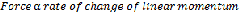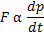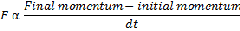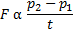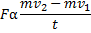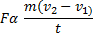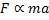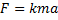If k = 1

Then this will reduce the equation to

F= ma

This where the famous equation F = ma come from. This why it is also considered as the Newton’s second law of motion